ConceptDraw
DIAGRAM 14

# UML Interaction Overview Diagram. Design Elements

UML Interaction Overview Diagram is a type of Interaction Diagrams that describes an overview in which the nodes represent interaction diagrams.

Interaction Overview Diagrams look the same as Activity Diagrams. The difference is that: on the Interaction Overview Diagram every individual activity describes as a frame that can include the nested Interaction Diagrams. This feature makes the Interaction Overview Diagrams useful to deconstruct the complex scenarios.

The Rapid UML Solution for ConceptDraw DIAGRAM contains 13 vector stencils libraries with 393 interactive shapes that you can use to design your UML diagrams.

To design an Interaction Overview Diagram use the UML Interaction Overview Diagram library.

UML Interaction Overview Diagram library contains 13 shapes:

• Interaction
• Initial node
• Final node
• Decision/merge node
• Parallel control flow
• Control flow
• Note
• Constraint
• Fork/join node
• Lifeline
• Smart connector
• Message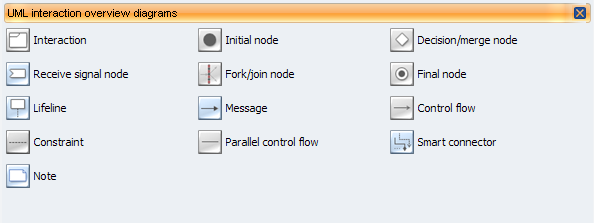Pic.1. UML Interaction Overview Diagram Library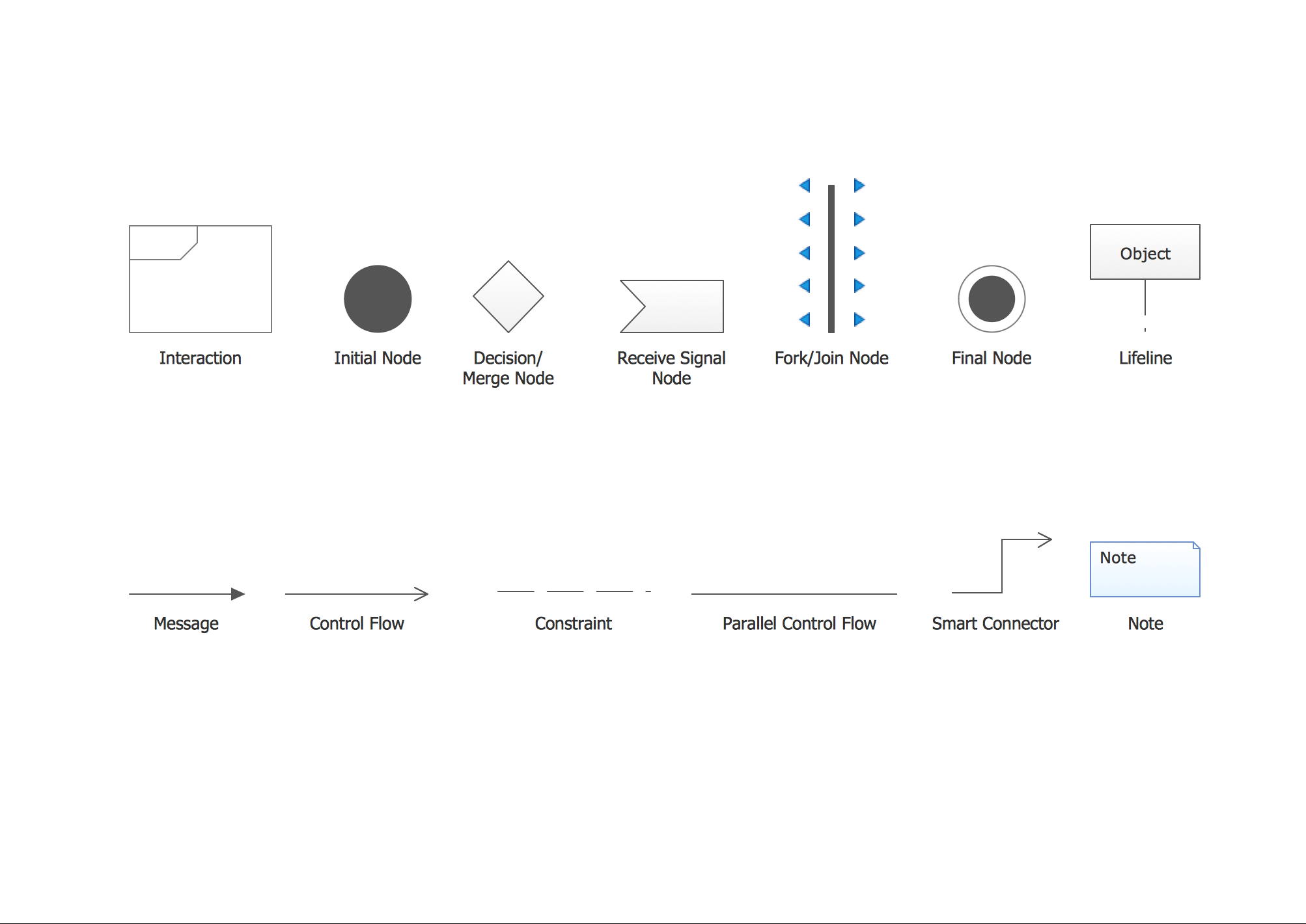Pic.2. UML Interaction Overview Diagram Library Elements

ConceptDraw Rapid UML solution provides UML Interaction Overview Diagram library of vector stencils for drawing the interaction overview diagrams using interaction overview blocks and assembly connectors.

All libraries for creating UML diagrams are available inside the ConceptDraw DIAGRAM Templates and samples are located in the Rapid UML section of ConceptDraw STORE.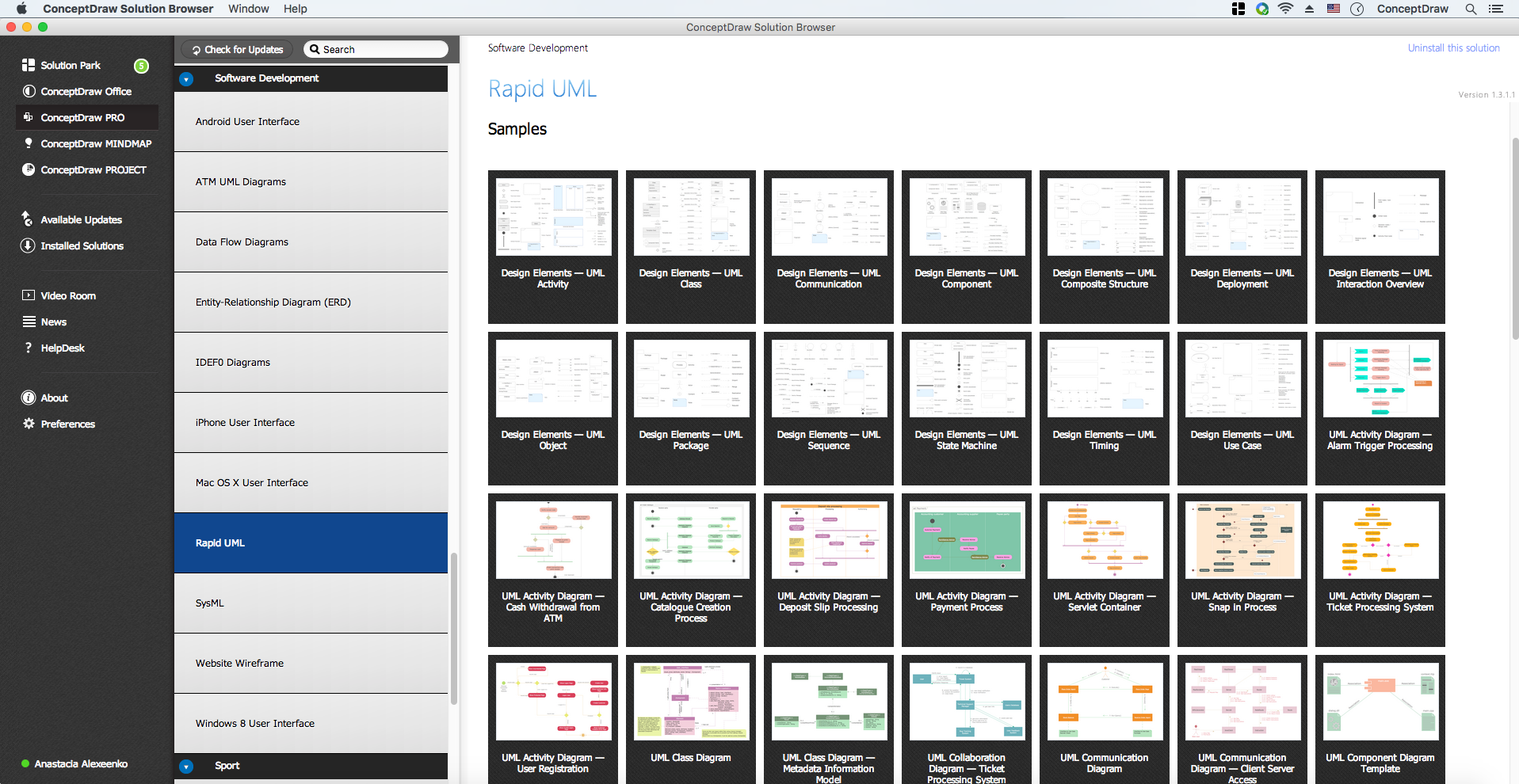Pic.3. UML Diagrams solution

Use design element from the UML Interaction Overview Diagram library to draw your own UML interaction overview diagrams of complex systems and software applications.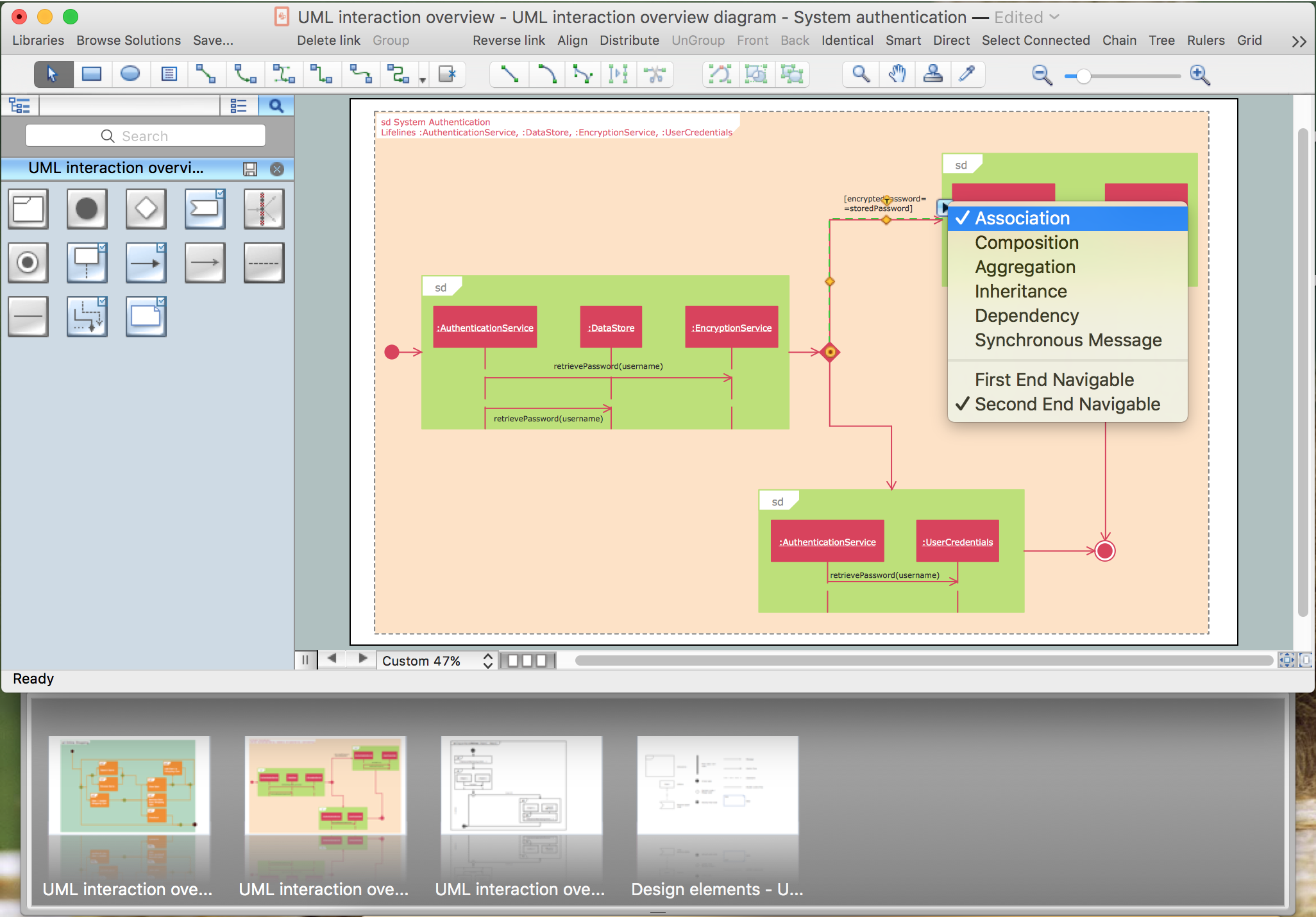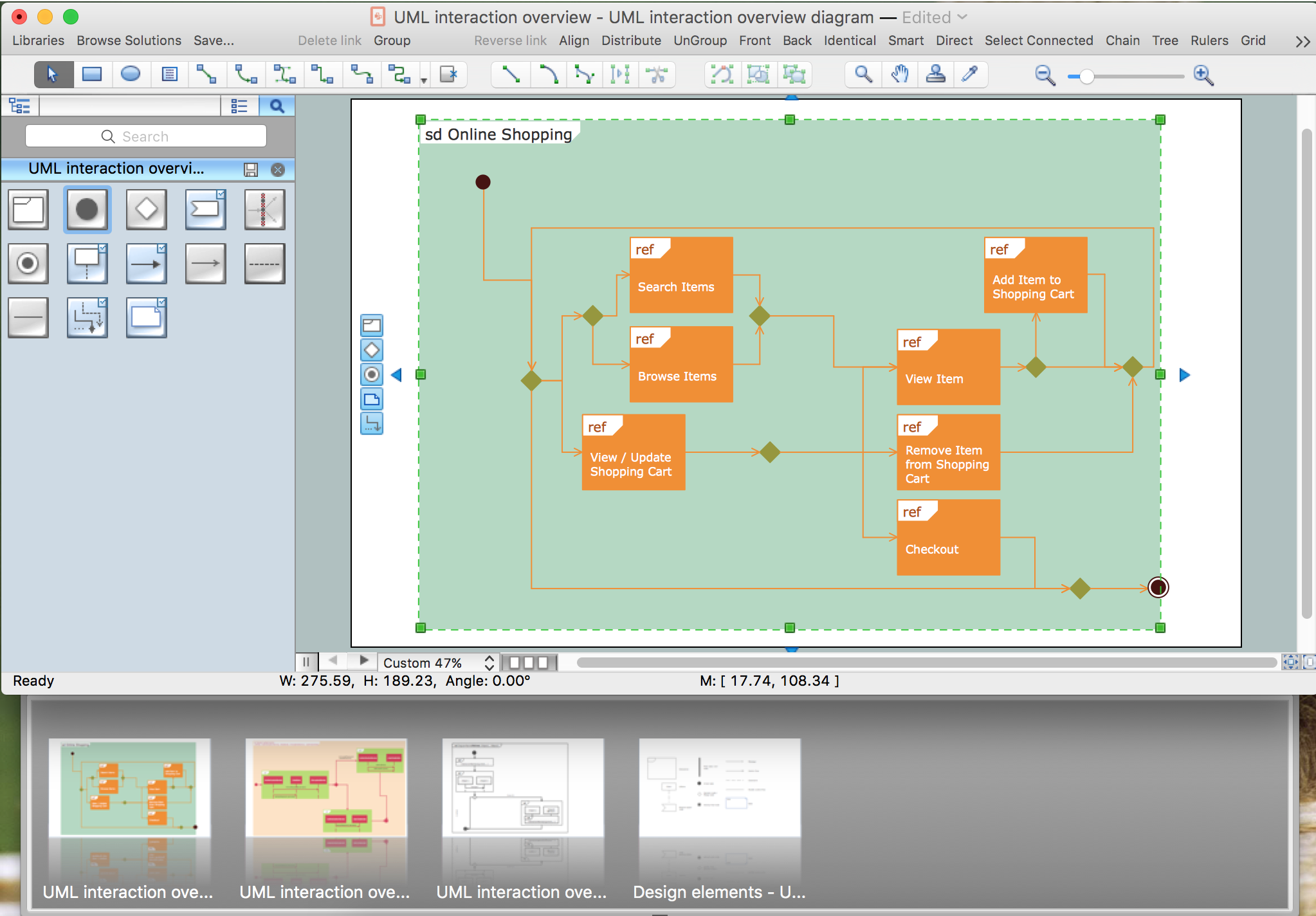### Introductory Guide to Rapid UML Solution →

The ability to develop UML diagrams as quickly as the ideas come. The solution uses ConceptDraw RapidDraw techniques. The result is a vector graphic document.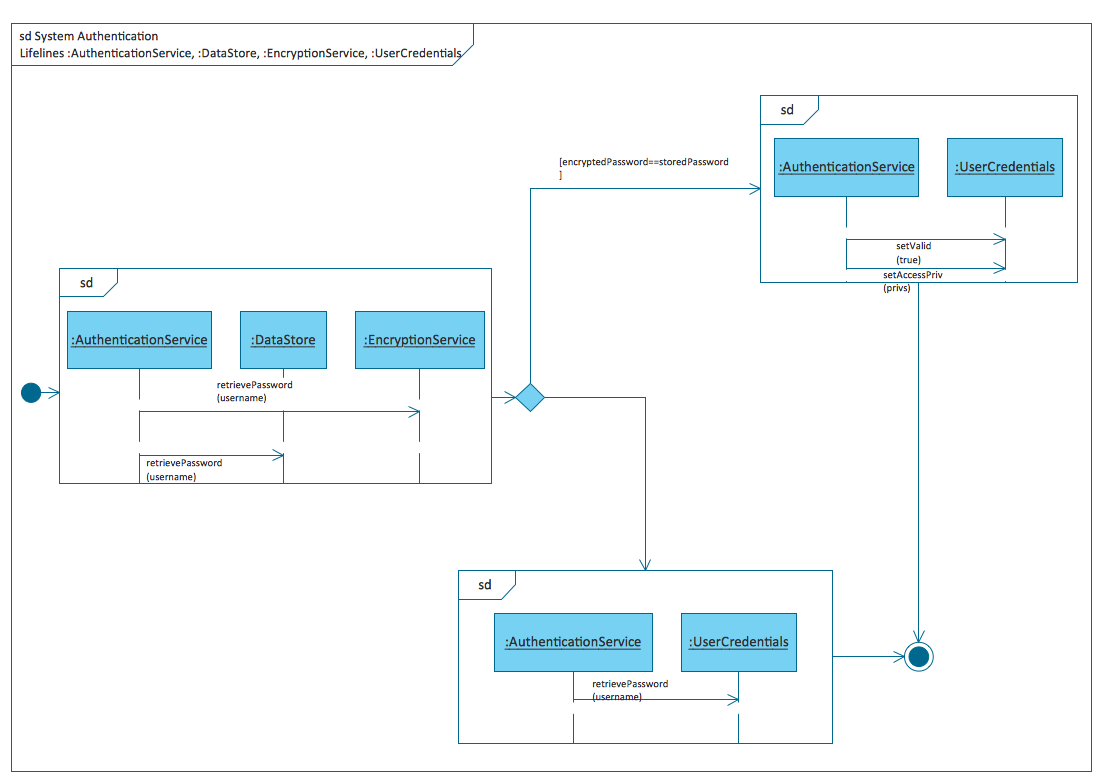Picture: Introductory Guide to Rapid UML Solution
Related Solution:

### Work Process →

Any work process visualized as a workflow diagram becomes easier-to-analyse. To visualize work processes regularly we recommend to use ConceptDraw DIAGRAM software extended with the Workflow Diagrams solution. This software contains features and technologies designed to draw workflow diagrams faster.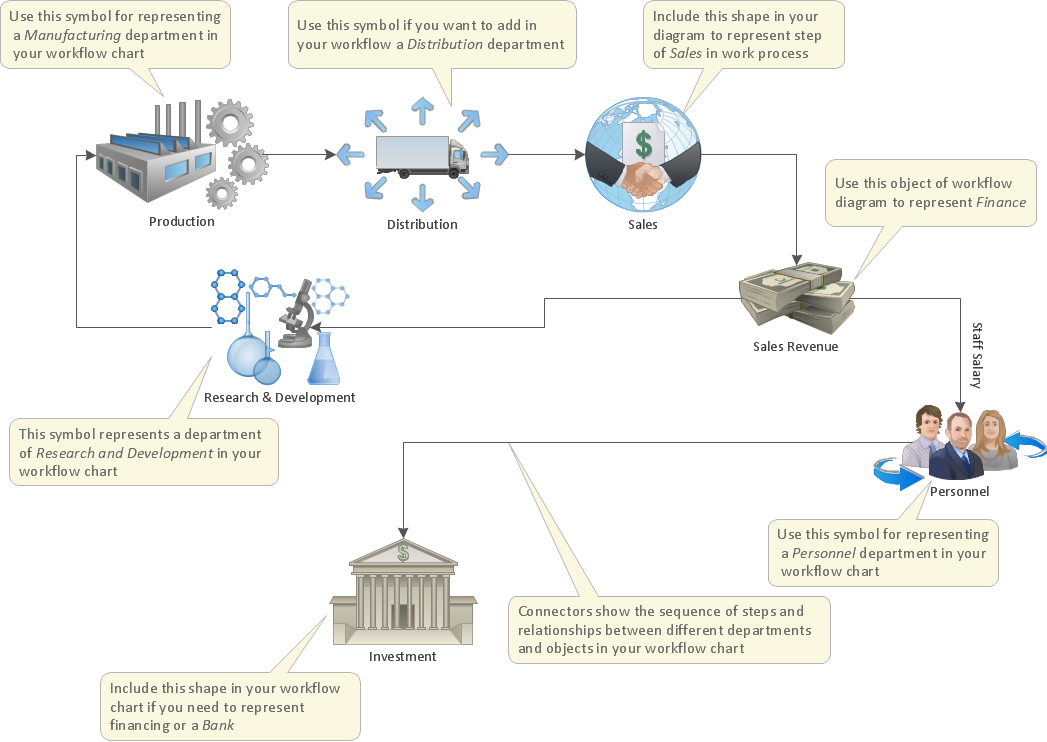Picture: Work Process
Related Solution:

### Software Diagram Examples and Templates →

ConceptDraw DIAGRAM is a powerful tool for business and technical diagramming. Software Development area of ConceptDraw Solution Park provides 5 solutions: Data Flow Diagrams, Entity-Relationship Diagram (ERD), Graphic User Interface, IDEFO Diagrams, Rapid UML.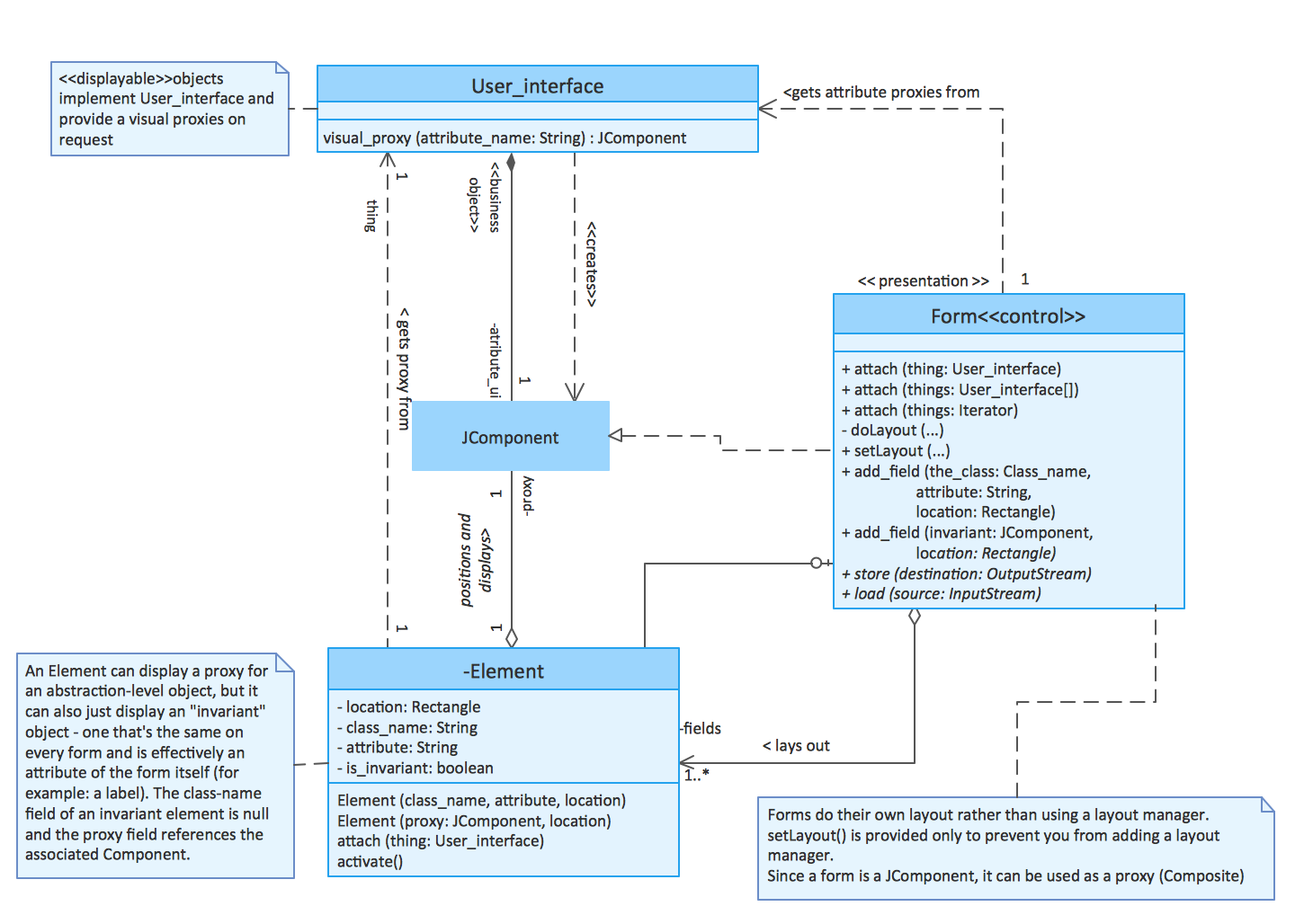Picture: Software Diagram Examples and Templates
Related Solution:

### Bank UML Diagram →

You need design a Bank UML Diagram? Now, thanks to the ConceptDraw DIAGRAM diagramming and vector drawing software extended with ATM UML Diagrams Solution from the Software Development Area, you can design without efforts any type of Bank UML Diagram you want - Class, Activity, Communication, Component, Composite structure, Deployment, Interaction overview, Object, Package, Profile, Sequence, State machine, Timing, Use case.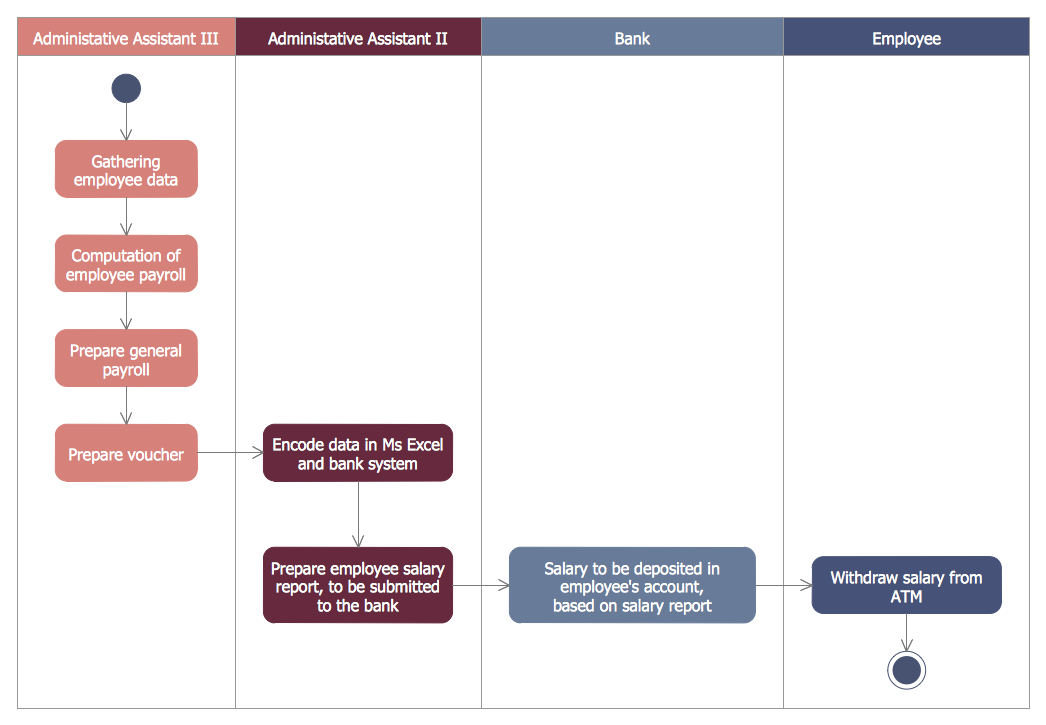Picture: Bank UML Diagram
Related Solution:

### Local area network (LAN). Computer and Network Examples →

Computer networks nowadays are spread all across the world. The large number of parameters, such as geographic scale or communication protocols, can divide networks. One of the most common types of networks is called local area network (LAN). It convenient to represent network examples by means of diagrams. This local area network (LAN) diagram provides an easy way to see the way the devices in a local network are interacted. The diagram uses a library containing specific symbols to represent network equipment , media and the end-user devices such as computers (PC, mac, laptop) , network printer, hubs, server and finally a modem. There are two types of network topologies: physical and logical. The current diagram represents precisely a physical type of LAN topology because it refers to the physical layout of a local network equipment.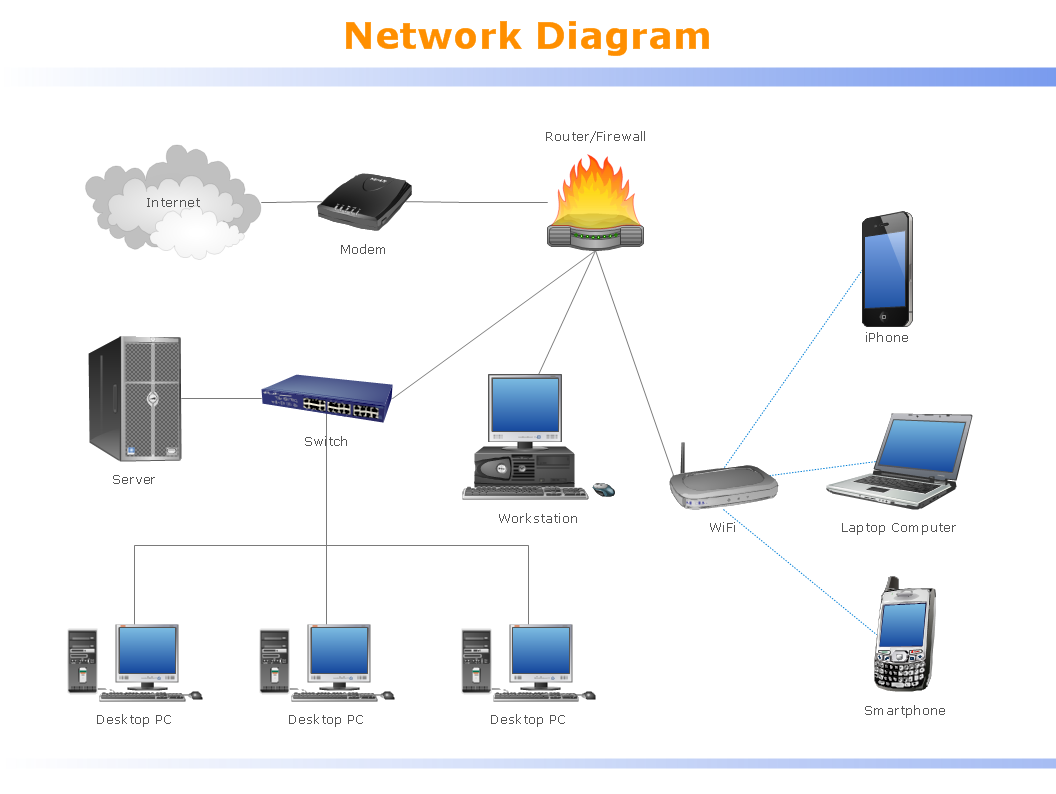Picture: Local area network (LAN). Computer and Network Examples
Related Solution:

### Data Flow Diagram Symbols. DFD Library →

Any information system receives data flows from external sources. In order to visualize them there is a list of data flow diagram symbols that describes how the system components cooperate. If you want to create a data flow diagram, ConceptDraw DIAGRAM Solution Park has DFD Library that contains both Yourdon and Gane-Sarson notations. This figure shows the content of vector libraries, delivered with ConceptDraw solution for data flow diagram (DFD). There are three libraries composed from about 50 vector objects used to make data flow diagrams. They include a complete set of objects utilized by Yourdon-Coad and Gane-Sarson notations - two primary notations that are apply for data flow diagramming. Also, one can discover additional "Data flow diagram (DFD)" library that provides a data flow diagram elements for designing level 1 and context-level data flow diagrams.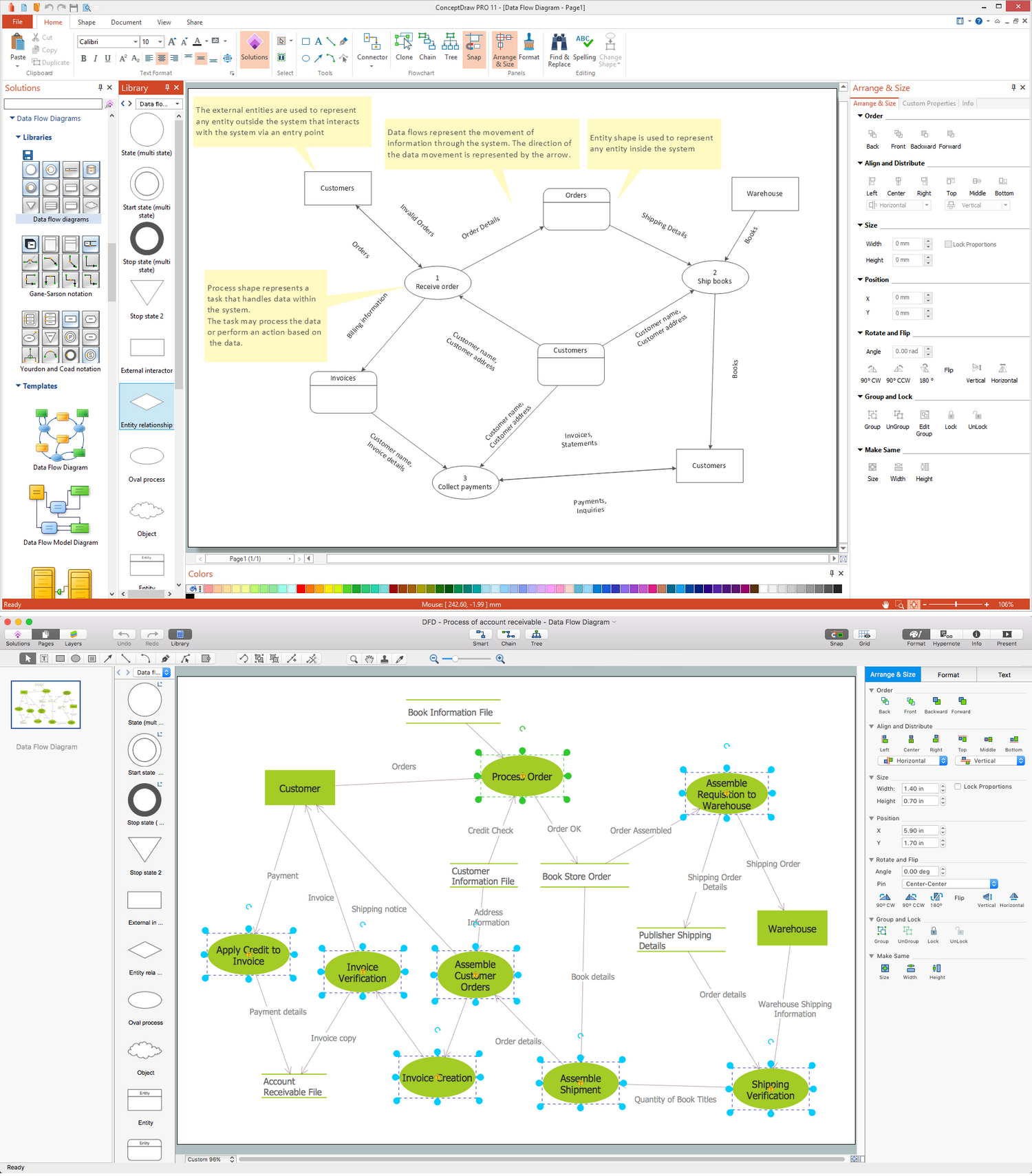Picture: Data Flow Diagram Symbols. DFD Library
Related Solution:
ConceptDraw
DIAGRAM 14